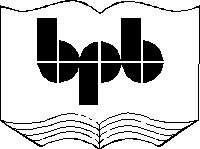15

# Unsafe code

Today, the programming language C is the most widely used because of only one reason and that is the use of pointers. In this chapter, we will explain what pointers are all about and how they can be used in the world of C#.

a.cs

class zzz

{

public static void Main()

{

yyy a = new yyy();

a.abc();

}

}

class yyy

{

public void abc()

{

int *i;

}

}

Compiler Error

a.cs(13,6): error CS0214: Pointers may only be used in an unsafe context

All simple variables like int, byte, short store numbers. When we create a variable in C#, we are allowed to put a multiplication/asterisk sign '*' in front of the variable. These variables are called Pointers. We get an error as C# considers pointers to be unsafe and hence we need special permission to use pointers.

a.cs

class zzz

{

public static void Main()

{

yyy a = new yyy();

a.abc();

}

}

class yyy

{

unsafe public void abc()

{

int *i;

}

}

Compiler Error

a.cs(11,20): error CS0227: Unsafe code may only appear if compiling with /unsafe

As seen in the previous chapter, the unsafe option must be tagged while compiling the program.  Give the command as csc a.cs /unsafe and the error now disappears.

a.cs

class zzz

{

public static void Main()

{

yyy a = new yyy();

a.abc();

}

}

unsafe class yyy

{

public void abc()

{

int *i;

}

}

The C# documentation very clearly states that the modifier can be used along with the class keyword, By using the modifier unsafe, we are asking C# to let us use pointers as we are unable to write code without the use of pointers.

a.cs

using System;

class zzz

{

public static void Main()

{

yyy a = new yyy();

a.abc();

}

}

class yyy

{

unsafe public void abc()

{

Console.WriteLine(sizeof(byte *) + " " + sizeof(short *) + " " + sizeof(int *) + " " + sizeof(long *));

Console.WriteLine(sizeof(byte **) + " " + sizeof(short **) + " " + sizeof(int **) + " " + sizeof(long **));

}

}

Output

4 4 4 4

4 4 4 4

Some time back, we had used the sizeof keyword, to determine how much memory C# allocates for our variables. When we ask for the sizeof an pointer variable, we always get 4, irrespective of whether the data type is a short or int or whatever, including another pointer.

a.cs

using System;

class zzz {

public static void Main() {

yyy a = new yyy();

a.abc();

}

}

class yyy {

unsafe public void abc() {

int  *i;

int j=1;

i = &j;

Console.WriteLine((int)i);

*i = 10;

Console.WriteLine(j);

}

}

Output

1243472

10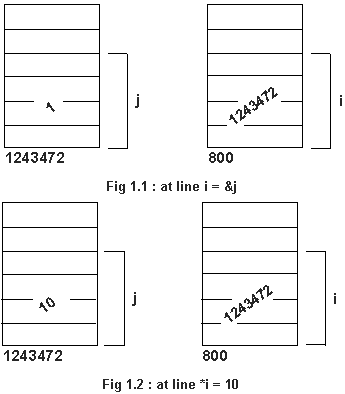We have created a variable j that has been initialized to one. We have also created a variable i, with a multiplication sign at the time of creation. By doing this C# allocated four memory locations to store i. What is most important is that a pointer variable is just like any another variable. It simply stores numbers. The single big difference is that a pointer variable value is interpreted as a computer memory location. If both i and j were being initialized to 1, then j is the number 1 as we know it, whereas i stand for computer memory location 1. Pointers can only be initialized to a computer memory location. Whenever we place an ampersand(&) in front of a variable, we are asking C# to tell us about the memory allocated for the variable. Every time C# creates a variable, it stores it somewhere in memory. We are at times interested in knowing where in memory the variable was allocated. A & in front of j will tell us the memory location where j starts in memory. As it is a computer memory location or address we can store it in i. C# allocated 4 memory location for j and the start of these 4 are being stored in a pointer.

We would like to display the value of the pointer. The WriteLine function does not have an overload to display a pointer and we have to cast it to an int. As we get 1243472 displayed, it could only mean that the variable j begins here and is spread over memory locations 1243473, 1243474 and 1243475.

We can only place the multiplication sign in front of a variable defined to be a pointer. If i is not a pointer, *i will return an error. C# here asks a simple question. What is the value of the variable i? . The answer is 1243472. C# will now go to memory location 1243472 to 1243475 and place the value 10 there. As the value of j is determined by what is present from memory locations 1243472 to 1243475, the value of j changes from 1 to 10.

a.cs

using System;

class zzz

{

public static void Main()

{

yyy a = new yyy();

a.abc();

}

}

class yyy

{

unsafe public void abc()

{

int  *i;

int j=1, k = 1;

i = &j;

Console.WriteLine((int)i);

*i = 10;

Console.WriteLine(j);

i = &k;

Console.WriteLine((int)i);

*i = 100;

Console.WriteLine(k + " " + j);

}

}

Output

1243468

10

1243464

100 10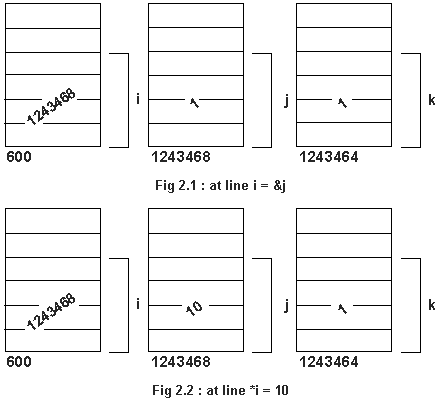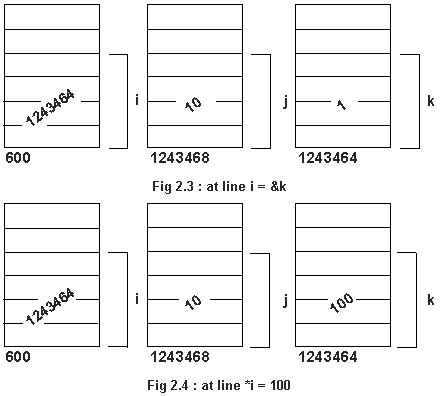Whenever we create a pointer variable, int *i, we are not stating explicitly which int i will be pointing to. Thus it can point to one int today, another int tomorrow. This is what gives pointers their flexibility. It can point to any int it wants to in memory. We are first initializing i to point to j, then we are changing the value of j to 10 through the pointer. Then i points to k in memory, and then k's value is indirectly being changed to 100 through the pointer. The first WriteLine tells us that the variable j begins at memory location 1243468. From here the next 4 have been reserved for the variable j. Memory for k has been allocated at 1243464. Thus, physically, k starts first in memory from 1243464 to 67 and j from 1243468 onwards.

a.cs

using System;

class zzz

{

public static void Main()

{

yyy a = new yyy();

a.abc();

}

}

class yyy

{

unsafe public void abc()

{

int  *i;

int j=1;

i = &j;

Console.WriteLine((int)i + " " + (int) &i);

}

}

Output

1243464 1243468

Lets not forget that pointers are also variables and C# allocates memory for i. An & in front of any variable tells us where it starts in memory. Thus i starts from memory location 1243468 onwards.

a.cs

using System;

class zzz

{

public static void Main()

{

yyy a = new yyy();

a.abc();

}

}

class yyy

{

unsafe public void abc()

{

long  *i;

long j=1, k = 1;

i = &j;

Console.WriteLine((int)i);

i = &k;

Console.WriteLine((int)i);

}

}

Output

1243460

1243468

The sizeof a long is 8 bytes and the addresses printed differ by 8 bytes.

a.cs

using System;

class zzz

{

public static void Main()

{

yyy a = new yyy();

a.abc();

}

}

class yyy {

unsafe public void abc(){

byte  *i;

byte j=1, k = 1;

i = &j;

Console.WriteLine((int)i);

i = &k;

Console.WriteLine((int)i);

}

}

Output

1243468

1243472

The only change here is that j and k are not longs but bytes. Why are we learning all about pointers is a question you should ask yourself. The C# documentation says very clearly that a byte occupies one memory location. The sizeof byte will also return one. In the above output, however, the memory locations differ by 4. The reason being that on the Pentium Processor, if we want one memory location, we will receive a minimum of 4. That's why, the Pentium is said to be a 32-bit processor. It will give you memory in chunks of 4. From this knowledge, using a byte instead of a int does not conserve memory or speed up your program. The above explanation cannot be comprehended without the knowledge of pointers.

a.cs

using System;

class zzz

{

public static void Main()

{

yyy a = new yyy();

a.abc();

}

}

class yyy

{

unsafe public void abc()

{

byte *i;

i = 0;

Console.WriteLine((int)i);

}

}

Compiler Error

a.cs(15,5): error CS0029: Cannot implicitly convert type 'int' to 'byte*'

C# is a strongly typed language and we are not allowed to convert a zero which is an int to a byte *, the data type of i. The only way out is to use a cast.

a.cs

using System;

class zzz

{

public static void Main()

{

yyy a = new yyy();

a.abc();

}

}

class yyy

{

unsafe public void abc()

{

byte *i;char *j;int *k;long *l;

i = (byte *)0; j = (char *)0; k = (int *)0 ; l = (long *)0;

Console.WriteLine((int)i + " " + (int) j + " " + (int) k + " " + (int)l);

i++;j++;k++;l++;

Console.WriteLine((int)i + " " + (int) j + " " + (int) k + " " + (int)l);

i++;j++;k++;l++;

Console.WriteLine((int)i + " " + (int) j + " " + (int) k + " " + (int)l);

}

}

Output

0 0 0 0

1 2 4 8

2 4 8 16

A pointer to any data type is allocated four memory locations at the time of creation. This enables pointers to store values that range from 0 to 4 billion. The question that comes to your mind, is what is the difference between a pointer to an int from a pointer to a long. We have created four variables i, j, k, l. Each is a pointer to a different data type. We have also initialized each variable to 0 and displayed their values using the WriteLine function. We have then incremented each of them by 1. To our surprise the pointer to an int k, increases by 4 and not by 1. The char pointer increases by 2 and long by 8. To make sure that is not a isolated phenomena, we increment them again. Same answer once again. They do not increase by one but by the size of the data type they point to. The sizeof a long is 8 and thus a pointer to a long increases by 8 and not 1.

When we initialized l to zero, we were telling C# that a long begins at memory location 0. This long will occupy the next 8 memory locations, form 0 to 7. Thus when we write l++, we are telling C# to take us to the next long in memory, which has to begins now at 8. The first difference between pointers to different data types is that the amount the pointer value increases is dependent upon what it is pointing to.

a.cs

using System;

class zzz

{

public static void Main()

{

yyy a = new yyy();

a.abc();

}

}

class yyy

{

unsafe public void pqr(int x , int y , int z)

{

int *p1; int *p2; int *p3;

p1 = &x; p2 = &y  ; p3 = &z;

Console.WriteLine((int) p1  + " " + (int) p2  + " " + (int) p3);

*p1 = 20;

*p2 = 30;

}

unsafe public void abc()

{

int *i ; int  *j ; int *k;

int l=1,m=2,n=3;

i=&l; j=&m; k=&n;

Console.WriteLine((int) i + " " + (int) j + " " + (int) k);

pqr(l,m,n);

Console.WriteLine(l + " " +  m + " " +  n); }}

Output

1243460 1243456 1243452

1243424 1243436 1243432

1 2 3

We have created a variable i of type int in memory. It begins at memory location 1243460. Similarly m and n start at 56 and 52 respectively. We are then calling a function pqr and passing three parameters to it. These are being stored at memory locations 1243424, 36 and 32 respectively. When we write *p1, we are going to memory location 1243424 and writing 20 there. This will change the value of x from 1 to 20. As variable l begins at 1243460, its value remains unchanged. The area of memory where x, y and z start is called the stack. Stack memory is used to pass parameters to functions.

a.cs

using System;

class zzz

{

public static void Main()

{

yyy a = new yyy();

a.abc();

}

}

class yyy

{

unsafe public void pqr(int x , int y , int z)

{

int *p1; int *p2; int *p3;

p1 = &x; p2 = &y  ; p3 = &z;

Console.WriteLine((int) p1  + " " + (int) p2  + " " + (int) p3);

}

unsafe public void xyz(int x , int y , int z)

{

int *p1; int *p2; int *p3;

p1 = &x; p2 = &y  ; p3 = &z;

Console.WriteLine((int) p1  + " " + (int) p2  + " " + (int) p3);

}

unsafe public void abc()

{

int *i ; int  *j ; int *k;

int l=1,m=2,n=3;

i=&l; j=&m; k=&n;

Console.WriteLine((int) i + " " + (int) j + " " + (int) k);

pqr(l,m,n);

xyz(l,m,n);

}

}

Output

1243460 1243456 1243452

1243424 1243436 1243432

1243424 1243436 1243432

Now you will begin to comprehend the importance of pointers. Its is an understanding of pointers that will give you a better insight into understanding the innards of a programming language. The last two WriteLines display the same answer as the stack memory gets reused for every function call. The stack is an area of memory that will store parameters and variables created in a function. Let us assume that the stack begins at memory location 100. The parameters are first pushed onto the stack and then all the local variables are created later, but below the parameters. Remember that the stack grows down in memory. When the function is over, the stack is moved back to 100, and the next function that gets called, reuses the same memory from 100. Thus anything created in a function has a lifetime of the open and close braces as the next function uses the same memory and the earlier values get overwritten.

a.cs

using System;

class zzz

{

public static void Main()

{

yyy a = new yyy();

a.abc();

}

}

class yyy

{

unsafe public void xyz()

{

int i=0,j=0,k=0;

int *p1; int *p2; int *p3;

p1 = &i; p2 = &j  ; p3 = &k;

Console.WriteLine((int) p1  + " " + (int) p2  + " " + (int) p3);

}

unsafe public void aaa()

{

int x=0,y=0,z=0;

int *p1; int *p2; int *p3;

p1 = &x; p2 = &y  ; p3 = &z;

Console.WriteLine((int) p1  + " " + (int) p2  + " " + (int) p3);

}

unsafe public void pqr()

{

int i=0,j=0,k=0;

int *p1; int *p2; int *p3;

p1 = &i; p2 = &j  ; p3 = &k;

Console.WriteLine((int) p1  + " " + (int) p2  + " " + (int) p3);

}

unsafe public void abc()

{

pqr();

xyz();

aaa();

}

}

Output

1243460 1243464 1243468

1243460 1243464 1243468

1243460 1243464 1243468

The above program proves a number of points. C# reuses the same memory for passing parameters and creating variables in functions. The variable names are not important as they get created on the stack, at the same place in memory.

a.cs

using System;

class zzz

{

public static void Main()

{

yyy a = new yyy();

a.abc();

}

}

class yyy

{

unsafe public void pqr(int x , int y , int z)

{

int *p1; int *p2; int *p3;

p1 = &x; p2 = &y  ; p3 = &z;

Console.WriteLine((int) p1  + " " + (int) p2  + " " + (int) p3);

p3++;p3++;p3++;p3++;p3++;p3++;

Console.WriteLine((int) p3);

*p3=20;

p3++;

Console.WriteLine((int) p3);

*p3=200;

}

unsafe public void abc()

{

int *i ; int  *j ; int *k;

int l=1,m=2,n=3;

i=&l; j=&m; k=&n;

Console.WriteLine((int) i + " " + (int) j + " " + (int) k);

pqr(l,m,n);

Console.WriteLine(l + " " +  m + " " +  n);

}

}

Output

1243460 1243456 1243452

1243424 1243436 1243432

1243452

1243456

1 200 20

Pointers are really unsafe. There is a simple rule in C# which says that variables created in one function abc, cannot be changed by another function pqr. The variables m and n begin at memory locations 1243456 1243452 respectively. If there was some way, I could write to these memory locations, I would be changing the values of m and n respectively. The pointer p3 stores, where the parameter z is stored in memory i.e. 1243432. If we can increment this pointer 6 times, 6 will actually be 24 due to pointer arithmetic, the value of p will now be 1243452 which is the address of n in memory. Thus from one function, I can change the value of another variable. In this case we are doing it on purpose but if I called a function, where a pointer went haywire, my variables change and nobody knows why the program stops working. Thus pointers are like a sharp knife, it can save a life in the hands of a doctor but in the wrong hands it can also kill.

a.cs

using System;

class zzz

{

public static void Main()

{

yyy a = new yyy();

a.abc();

}

}

class yyy

{

unsafe public void pqr(int x , int y , int z)

{

int *p1; int *p2; int *p3;

p1 = &x; p2 = &y  ; p3 = &z;

Console.WriteLine((int) p1  + " " + (int) p2  + " " + (int) p3);

int q = 1;

while ( q <= 12 )

{

p1++;

Console.WriteLine((int) p1 + " " + *p1);

q++;

}

}

unsafe public void abc()

{

int *i ; int  *j ; int *k;

int l=1,m=2,n=3;

i=&l; j=&m; k=&n;

Console.WriteLine((int) i + " " + (int) j + " " + (int) k);

pqr(l,m,n);

Console.WriteLine(l + " " +  m + " " +  n);

}

}

Output

1243460 1243456 1243452

1243424 1243436 1243432

1243428 47645012

1243432 3

1243436 2

1243440 0

1243444 1243508

1243448 1243544

1243452 3

1243456 2

1243460 1

1243464 1243452

1243468 1243460

1243472 1243484

1 2 3

Would it not be a great idea to be able to display whatever is there on the stack. Between variables there are some gaps and the above program is displaying the contents of the stack. The variable q makes the loop go on 12 times and each time we increment p1 by 4. P1 is pointing to x the first parameter on the stack. Would it not aid understanding if Microsoft explained what all it pushes on the stack. One of the most common hacking exploits on the net is called a stack overflow.

a.cs

using System;

class zzz

{

public static void Main()

{

yyy a = new yyy();

a.abc();

}

}

public class yyy

{

unsafe public void pqr( int *a)

{

Console.WriteLine("{0} {1} {2}",a,a,a);

}

unsafe public void abc()

{

int [] a = new int;

a = 10; a = 2; a = 30;

pqr(a);

}

}

Compiler Error

a.cs(20,1): error CS1502: The best overloaded method match for 'yyy.pqr(int*)' has some invalid arguments

a.cs(20,5): error CS1503: Argument '1': cannot convert from 'int[]' to 'int*'

We were trying to pass an array as a parameter to a function. Unfortunately C# has some other views.

a.cs

using System;

class zzz

{

public static void Main()

{

yyy a = new yyy();

a.abc();

}

}

public class yyy

{

unsafe public void pqr( int *a)

{

Console.WriteLine("{0} {1} {2}",a,a,a);

}

unsafe public void abc()

{

int [] a = new int;

a = 10; a = 2; a = 30;

fixed ( int *i = a) pqr(i);

}

}

Output

10 2 30

The keyword fixed removed the error. An array in memory can be moved around by C#. We would like C# to keep it fixed in memory. The keyword fixed guarantees that C# will not move it around in RAM for the duration of the program. The only reason C# moves objects in memory is to speed up execution of the program. The keyword fixed as part of syntax wants the () .Within them you can create a variable in our case i which we initialize to a C# object, an array a. The scope or lifetime of this array is in the next statement i.e. the invocation of function pqr. In case you have more lines you can use the {}. Even though a now is a pointer to an int, the notation a refers to the first member. The name of an array tells us where the array starts in memory.

a.cs

using System;

class zzz

{

public static void Main()

{

yyy a = new yyy();

a.abc();

}

}

public class yyy

{

unsafe public void pqr( int *a)

{

Console.WriteLine("{0} {1} {2}",a,a,a);

a = 100 ; a = 40; *(a+2) = 33;

}

unsafe public void abc()

{

int [] a = new int;

a = 10; a = 2; a = 30;

fixed ( int *i = a) pqr(i);

Console.WriteLine("{0} {1} {2}",a,a,a);

}

}

Output

10 2 30

100 40 33

Changing anything through a pointer changes the original. Whenever we write a= , we are changing the first member of the original array. a is only a notation, it actually get converted to *(a+1). Thus we are changing the original members of the array. Also the array grows upward in memory.

a.cs

using System;

class zzz

{

public static void Main()

{

yyy a = new yyy();

a.abc();

}

}

public class yyy

{

unsafe public void pqr( int *a)

{

Console.WriteLine("{0} {1} {2}",a,a,a);

for ( int i = 0 ; i<= 2000; i++)

*(a+i) = i*10;

}

unsafe public void abc()

{

int [] a = new int;

a = 10; a = 2; a = 30;

fixed ( int *i = a) pqr(i);

Console.WriteLine("{0} {1} {2}",a,a,a);

}

}

Please do not run the above program as the error would be unpredictable. The reason being that we are writing beyond the bounds of the array. The array a created in the function abc has only 12 memory locations allocated for it. We are writing the next 2000 * 4, 8000 memory locations. The error on your machine will be very different form another machine. This unpredictability is why pointer errors are hard to catch, and your boss would thus not like you to use a pointer while programming.

a.cs

using System;

class zzz

{

public static void Main()

{

yyy a = new yyy();

a.abc();

}

}

struct aaa

{

public int i,j;

}

class yyy

{

unsafe public void abc()

{

aaa *a;aaa b;

b = new aaa();

a = (aaa *)b;

}

}

Compiler Error

a.cs(20,10): error CS0030: Cannot convert type 'aaa' to 'aaa*'

aaa is a structure with two members i and j. new aaa() allocates memory for i and j. new aaa() and b are of the same data type ie aaa. When we try to equate a and b, C# canot convert a pointer to a struct/class to the object itself. Remember new returns not a pointer to an object but the object itself.

a.cs

using System;

class zzz

{

public static void Main()

{

yyy a = new yyy();

a.abc();

}

}

struct aaa

{

public int i,j;

}

class yyy

{

unsafe public void abc()

{

aaa *a;aaa b;

b = new aaa();

a = &b;

int *x;

x = &(a->i);

int *y;

y = &(a->j);

Console.WriteLine((int) a + " " + (int)x + " " + (int) y);

}

}

Output

1243468 1243468 1243472

We need to take the address of where b begins in memory by using the & and equate that to a. The address of an object like aaa is of the data type aaa *. As a now is a pointer to a structure, we have to use a different syntax to display the members of the structure. The new way is to use the -> operator. Thus a->i refers to the member i of the structure that looks like aaa. The variable x now stores the address of where the first member i begins in memory. Obviously the address of the first member i and the address of the structure will be the same and the second member will be stored 4 later.

a.cs

using System;

class zzz

{

public static void Main()

{

yyy a = new yyy();

a.abc();

}

}

struct aaa

{

public int i,j;

}

class yyy

{

unsafe public void abc()

{

aaa *a;aaa b;

b = new aaa();

a = &b;

Console.WriteLine((int) a);

b = new aaa();

a = &b;

Console.WriteLine((int) a);

}

}

Output

1243468

1243468

We have one object b that we have initialized twice with new. Each time we get the same memory location as the address of b in memory will remain the same.

Unions

a.cs

using System.Runtime.InteropServices;

class zzz

{

unsafe public static void Main()

{

yyy a = new yyy();

a.i = 65536+512+3;

System.Console.WriteLine(a.i + " " + a.j + " " + a.k);

int *i1;short *j1;byte *k1;

i1 = &a.i; j1 = &a.j ; k1 = &a.k;

System.Console.WriteLine((int)i1 + " " + (int) j1 + " " + (int) k1);

System.Console.WriteLine(sizeof(yyy));

}

}

[StructLayout(LayoutKind.Explicit)]

struct yyy

{

[FieldOffset(0)] public int i;

[FieldOffset(0)] public short j;

[FieldOffset(0)] public byte k;

}

Output

66051 515 3

1243476 1243476 1243476

4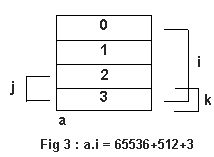Let us understand what a union is all about. We are printing the addresses of i, j and k and lo and behold they are all the same. In a union all the members begin at the same place and that is why their addresses were similar. If we understand pointers, understanding unions then becomes a piece of cake as we can visually see the addresses.

a.cs

using System.Runtime.InteropServices;

class zzz

{

unsafe public static void Main()

{

yyy a = new yyy();

a.i = 65536+512+3;

System.Console.WriteLine(a.i + " " + a.j + " " + a.k);

int *i1;short *j1;byte *k1;

i1 = &a.i; j1 = &a.j ; k1 = &a.k;

System.Console.WriteLine((int)i1 + " " + (int) j1 + " " + (int) k1);

System.Console.WriteLine(sizeof(yyy));

}

}

[StructLayout(LayoutKind.Explicit)]

struct yyy

{

[FieldOffset(0)]

public int i;

[FieldOffset(0)]

public short j;

[FieldOffset(10)]

public byte k;

}

Output

66051 515 0

1243468 1243468 1243478

11

a.cs

using System;

class zzz

{

public static void Main()

{

yyy a = new yyy();

a.abc();

}

}

class yyy

{

unsafe public void abc()

{

short i = 512+3;

byte *j;

j = (byte *)&i;

Console.WriteLine((int)j + " " + i );

*j = 1;

Console.WriteLine(i);

j++;

Console.WriteLine((int)j);

*j=1;

Console.WriteLine(i);

j++;

Console.WriteLine((int)j);

*j=10;

Console.WriteLine(i);

}

}

Output

1243468 515

513

1243469

257

1243470

257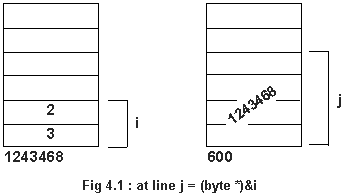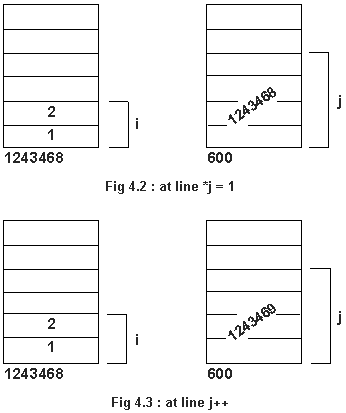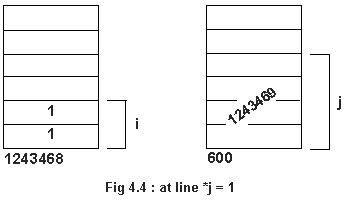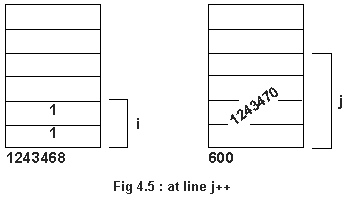j is a pointer to a byte. From the output, we can see that i begins at 1243468. i is of data type short which means it takes 2 bytes. j now contains the the address value of i i.e. 1243468. The first line proves it. The statement *j=1 will change the bottom byte value to 1.j++ will then increment the address value by 1, hence *j=1 will now change the top byte to 1. The last j++ has no effect because the scope of short is only 2 btes. Hence we see no change in the value of i.

Here, in place of the data type short, we have given an int.

a.cs

using System;

class zzz {

public static void Main()

{

yyy a = new yyy();

a.abc();

}

}

class yyy {

unsafe public void abc()

{

int i = 512+3;

byte *j;

j = (byte *)&i;

Console.WriteLine((int)j + " " + i );

*j = 1;

Console.WriteLine(i);

j++;

Console.WriteLine((int)j);

*j=1;

Console.WriteLine(i);

j++;

Console.WriteLine((int)j);

*j=10;

Console.WriteLine(i);

}

}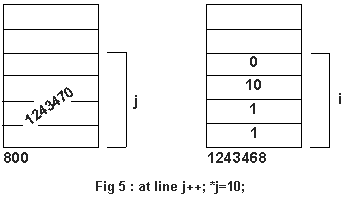Output

1243468 515

513

1243469

257

1243470

655617

The last j++ in the series will touch the third byte, there by replacing the 1 to 10. Hence the output changes dramatically.